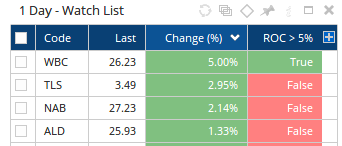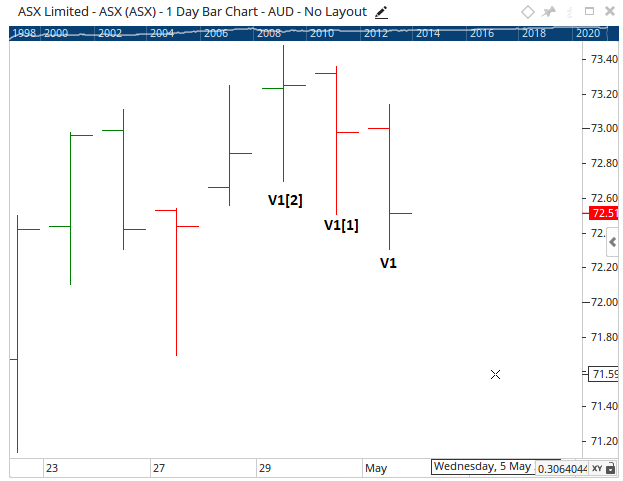Select Page

# Bullish Combination Scan

Optuma Forums Optuma Scripting Bullish Combination Scan

Viewing 8 posts - 1 through 8 (of 8 total)
• Author
Posts
• #63733

Hi everyone,

I’m new to Optuma scripting and am hoping if someone can see where I’m going wrong here.

Attempting to write a script for either a % breakout or a \$ breakout, I’ve come up with the following.

(CLOSE()-OPEN()>=.90 and VOL()>100000 and CLOSE()-OPEN() < CLOSE() – OPEN() and CLOSE()/CLOSE()<=1.02) or (CLOSE()/CLOSE()>=1.04 and VOL()>VOL() and VOL()>=100000 and CLOSE()/CLOSE()) <=1.02 and CLOSE()>3 and (CLOSE()-LOW())/(HIGH()-LOW())>.70

I’ve put this all as one function in the scanning manager so am not sure if that is suitable as a side note, but with regards to the results, I’m showing stocks with negative price action today, though this should be limited to the preceding days.

Another issue is that stocks are showing wild percentage changes as you can see from the attached screenshot.

Thank you.

#63756

Hi,

You can use functions like ROC() to find the % change from day to day, then setup a script to look for increases.

Something like this would work (using a 5% change as an example):If you wanted to make sure the previous day was not also a high % increase you could include the following:

The above would only trigger if the current bar had a Rate of Change higher than 5% but the previous bar was lower than 5%. You can use this same type of offset on the variable (V1) to compare bars going back further (V1 V1 etc).

If this isn’t what you are after please provide a screen shot example of the setup on a chart with more information and I can add additional details here.

#63757

Hi Matthew,

Thank you, that ROC seems like it would work well. How would it look like if I wanted to run that ROC function for days previous to today? For example yesterday from the day before.

Here is the detail behind what I was trying to achieve in the whole scan.

Thank you.

Bullish Combination Scan
(CLOSE()-OPEN()>=.90 and VOL()>100000 and (CLOSE()-OPEN() < CLOSE() – OPEN()) and CLOSE()/CLOSE()<=1.02) or (CLOSE()/CLOSE()>=1.04 and VOL()>VOL() and VOL()>=100000 and CLOSE()/CLOSE()) <=1.02 and CLOSE()>=3 and (CLOSE()-LOW())/(HIGH()-LOW())>=.70

Today’s close minus today’s open is equal to or greater than 90c, and
Today’s volume greater than 100,000, and
Yesterday’s close minus yesterday’s open is less than today’s close minus today’s open, and
Yesterday’s close divided by the previous day’s close is equal to or less than 1.02
OR
Today’s close divided by yesterday’s close is greater than or equal to 1.04 and
Today’s volume is greater than yesterday’s volume, and
Today’s volume is greater than 100,000, and
Yesterday’s close divide by the previous day’s close is less than or equal to 1.02, and
Today’s close price is equal to or greater than \$3, and
Today’s close minus today’s low divided by today’s high minus today’s close is equal to or greater than .70 (closing within 70% of high)

#63758

Hi,

Regarding referencing previous days values, the second script in my original reply shows how best to do this. You setup a variable (V1 for the ROC in this case) then use square brackets for the Offset.

V1 = Current Bar
V1 = Previous Bar
V1 = 3rd last bar
etcWith regards to your specific example, below is how i would script the top criteria:

Because we are dealing with a specific value range (rather than a %) the results are limited (37 results on the ASX exchange over the last quarter). I’ve attached a sample workbook with a list of the 37 codes that passed in the last quarter, with a Show Bar highlighting the bars that passed (red arrows) and the Analysis Cluster below with each rule broken into individual scripts.

You can see the red arrows (the trigger) pass when all 4 rules are met.

#63765

Thanks Matthew, apologies, I missed the second part of your post explaining the historical change.

I’ll play around with that now.

Jeremy

#63811

Rule 1

Today’s close minus today’s open is equal to or greater than 90c, and

Rule 2

Today’s volume greater than 100,000, and

Rule 3

Yesterday’s close minus yesterday’s open is less than today’s close minus today’s open, and

Rule 4

Yesterday’s close divided by the previous day’s close is equal to or less than 1.02

//Rule 1

COV = (Close() – Open());

V1 = COV >= 0.90;

//Rule 2

V2 = VOL() > 100000;

//Rule 3

V3 = COV > COV ;

//Rule 4

V4 = (CLOSE() / CLOSE(1)) <= 1.02 ;

//All Rules Pass

V1 and V2 and V3 and V4

It looks like the above rules are for the \$ breakout and once all four rules are met, then a stock will show in the scan results.

Should this be written as one large script first, then added into the scan manager?
Could you please clarify the COV function.
Rule 3 is yesterday’s close minus yesterday’s open is less than today’s close minus today’s open.
Should the rule not therefore be “V3 = COV<COV?
Rule 4 is yesterday’s close divided by the previous day’s close is equal to or less than 1.02.
Should the rule not therefore be “V4 = (CLOSE(1) / CLOSE (2)) <= 1.02?

To answer your previous question, I’m looking for a setup like the following…

Very tight range in the preceding days, then a breakout on the market open, either 4% plus, or \$1.

#63814

Hi,

Should this be written as one large script first, then added into the scan manager?
I am not sure what you mean. The script provided is a single script (just with multiple rules), it can be copied and used in the scanning manager as a whole.

Could you please clarify the COV function.
COV is not a function, it is the name of the variable (like V1, V2, etc) i setup for the difference between the Close and Open of a bar.  So C = 2 and O = 1.5 the COV value would be 0.5

Rule 3 is yesterday’s close minus yesterday’s open is less than today’s close minus today’s open.
Should the rule not therefore be “V3 = COV<COV?
Yes swap > to < if it needs to be less than.

Rule 4 is yesterday’s close divided by the previous day’s close is equal to or less than 1.02.
Should the rule not therefore be “V4 = (CLOSE(1) / CLOSE (2)) <= 1.02?
Yes, if it is the previous bars close being compared to the bar before it your adjustment is right.

#63833

Hi Matthew,

I’ve continued to work on getting this scan up and running and have outlined what I have so far including the description of the rules.

90c Breakout Script

Rule 1: today’s (current) close price is greater than 90c on open price
Rule 2: Today’s volume >100,000)
Rule 3: Yesterday’s close-open variance is less than today’s close-open variance)
Rule 4: Yesterday’s close divided by the previous day’s close is less than or = 1.02)

//Rule 1
COV = (Close() – Open());
V1 = COV >= 0.90;
//Rule 2
V2 = VOL() > 100000;
//Rule 3
V3 = COV()<COV();
//Rule 4
V4 = (CLOSE() / CLOSE()) <= 1.02;
//All Rules Pass
V1 and V2 and V3 and V4

The scan results show zero stocks though this morning on TC2000, this scan picked up 453 stocks from the NASDAQ/NYSE. I appreciate that this is a larger universe, though the scan criteria to me doesn’t seem overly restrictive so I am curious about the lack of results on the ASX.

4% Breakout Script
I’ve had an attempt at part two of the scan which is the 4% breakout and have stipulated the following rules.

Rule 1: Today’s close divided by yesterday’s close is greater than or equal to 1.04 and
Rule 2: Today’s volume is greater than yesterday’s volume, and
Rule 3: Today’s volume is greater than 100,000, and
Rule 4: Yesterday’s close divide by the previous day’s close is less than or equal to 1.02, and
Rule 5: Today’s close price is equal to or greater than \$3, and
Rule 6: Today’s close minus today’s low divided by today’s high minus today’s close is equal to or greater than .70 (closing within 70% of high)

//Rule 1
V1 = ROC(BARS=1) > 4;
//Rule 2
V2 = VOL() > VOL());
//Rule 3
V3 = VOL>100000;
//Rule 4
V4 = (CLOSE() / CLOSE()) <= 1.02 ;
//Rule 5
V5 = CLOSE()=>3
//Rule 6
CLV = (Close() -Low ());
HLV = (High()-Low())>.7
V6 = (CLV()/(HLV())>.70
//All Rules Pass
V1 and V2 and V3 and V4 and V5 and V6

The script isn’t valid so I’m missing something here. If you could point out where I’m going wrong that would be great.

Jeremy

Viewing 8 posts - 1 through 8 (of 8 total)
• You must be logged in to reply to this topic.